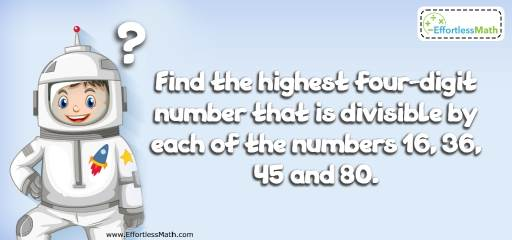# Other Topics Puzzle – Challenge 99

This cool puzzle (and solution) can help you challenge your students. It only requires basic knowledge of mathematics. Let's see who can solve it!## Challenge:

Find the highest four-digit number that is divisible by each of the numbers 16, 36, 45 and 80.

### The Absolute Best Book to challenge your Smart Student!

For a positive integer to be divisible by those numbers, it must be divisible by their Least common multiple:
Factorize the numbers to find their Least Common Multiple.
$$16 = 2^4$$
$$36 = 2^2 × 3^2$$
$$45 = 3^2 × 5$$
$$80 = 2^4 × 5$$
Least Common Multiple $$= 2^4 × 3^2 × 5 = 720$$
Every multiple of 720 is divisible by all those above.
Every multiple of 720 can be written as 720n, where n is an integer. The largest 4-digit integer is 9999. Therefore:
$$720n ≤ 9999 → n ≤ \frac{9999}{720} → n ≤ 13.8875 → n = 13 → 720n = 9360$$

### What people say about "Other Topics Puzzle – Challenge 99 - Effortless Math: We Help Students Learn to LOVE Mathematics"?

No one replied yet.

X
30% OFF

Limited time only!

Save Over 30%

SAVE $5 It was$16.99 now it is \$11.99